# Review

## 1. 信息的表示和处理

• MSB：most significant bit（最高有效位）
• LSB：least significant bit（最低有效位）

### 1.1 进制表示

• 二进制数用后缀字母 B
• 十六进制数用后缀字母 H
• C 语言常量数字默认为有符号数，无符号数用后缀字母 U

• 整数转换

• 小数转换

### 1.3 数值范围

• 无符号数值

$UMin = 0 \\ UMax = 2^w - 1 \\$

• 补码数值

$TMin = -2^{w-1} \\ TMax = 2^{w-1} - 1 \\$

### 1.4 类型转换

• 有符号数和无符号数的转换规则：

#### 隐式转换

• 有符号数隐式转换为无符号数
当表达式中有符号和无符号数混用时，包括比较运算符连接的表达式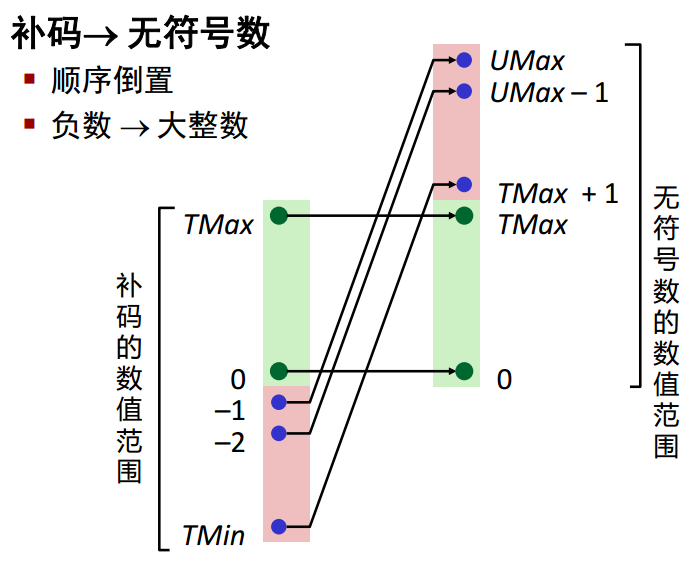#### 符号扩展

• 对于给定 w 位的有符号整型数 x 转为 w+k 位相同数值的整型数，将符号位复制 k 份
C 语言中从短整数类型向常整数类型转换时自动进行符号扩展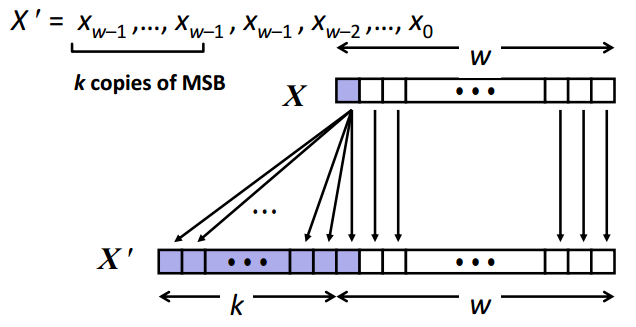#### 整数截断

• 无符号数的截断（w 位 $\rightarrow$ k 位）

$\begin{array}{c} X' = X \mod 2^k \end{array}$

• 有符号数的截断（w 位 $\rightarrow$ k 位）

$\begin{array}{c} X' = (signed)((unsigned)X \mod 2^k) \end{array}$

### 1.5 整数运算

• 加法

#### 1. 无符号数加法

$\begin{array}{c} UAdd_w(x,y) = (x + y) \mod 2^w = \begin{cases} x + y & x + y \lt 2^w \\ x + y - 2^w & x+y \geq 2^w \\ \end{cases} \end{array}$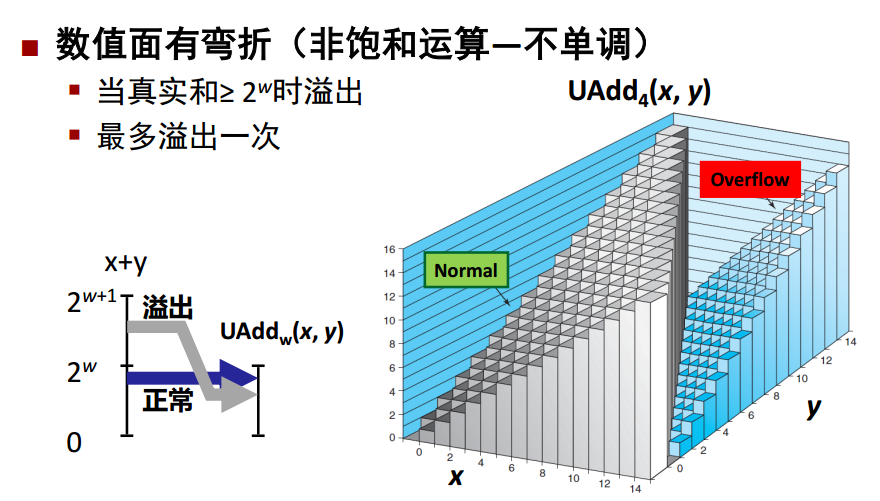#### 2. 有符号数加法

$\begin{array}{c} TAdd_w(x,y) = (signed)UAdd_w((unsigned)x,(unsigned)y) \\ = \begin{cases} x + y - 2^w & TMax_w \lt x + y \ \text{正溢出} \\ x + y & TMin_w \leq x + y \leq TMax_w \ \text{正常} \\ x + y + 2^w & x + y \lt TMin_w \ \text{负溢出} \end{cases} \end{array}$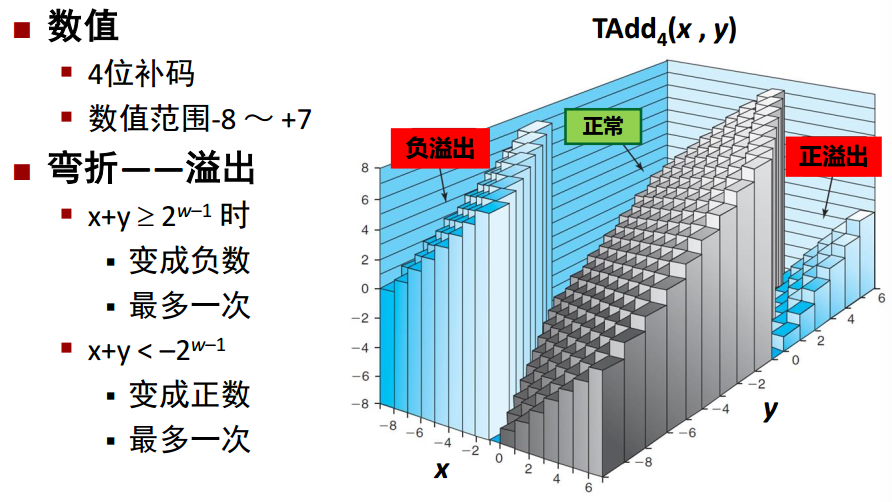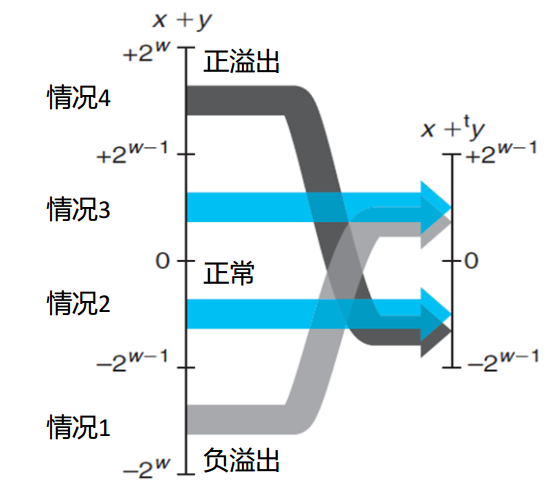【注】CPU 其实并不知道操作的是有/无符号数，CPU 所做的便是将两个 w 位的二进制数 x、y 相加并将结果的进位 w+1 位去掉（即只保留结果的后 w 位）。

• 乘法

$\begin{array}{c} UMult_w(x,y) = (x \cdot y) \mod 2^w \\ TMult_w(x,y) = (signed)UMult_w((unsigned)x,(unsigned)y) \\ \end{array}$

• 除法
整数除法遵循向零舍入的原则，即：

$\begin{array}{c} TDiv(x,y) = \begin{cases} \lfloor {x \over y} \rfloor & x \cdot y \gt 0 \\ 0 & x = 0 \\ \text{错误} & y = 0 \\ \lceil {x \over y} \rceil & x \cdot y \lt 0 \\ \end{cases} \end{array}$

#### 1. 向上舍入转为向下舍入：

$\begin{array}{c} \lceil {x \over y} \rceil = \lfloor {x + y - 1 \over y} \rfloor \end{array}$

#### 2. 使用移位表示 2 的整数幂除法

$\begin{array}{c} {x \over 2^k} = (x \lt 0 \ ? \ x+(1 \ll k)-1 : x) \gg k \end{array}$

## 2. 程序的机器级表示

### 2.1 计算机系统中的抽象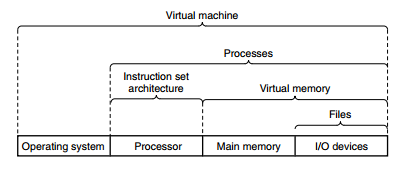### 2.2 操作数类型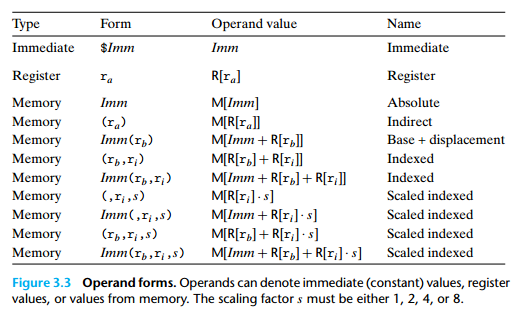### 2.3 指令

x86 汇编语言有两种语法：AT&T 、Intel 。其中前者用于 Unix 系统家族，后者用于 DOS 和 Windows 家族。这两种语法格式的区别如下：

mov $5, %eax 源操作数在目的操作数的右边 mov eax, 5 操作数大小 指令有后缀，且后缀指定的大小和给出的操作数大小要匹配 addl$4, %esp

add esp, 4

add esp, 4

movl mem_location(%ebx,%ecx,4), %eax
【注】此时立即数前不需要加 \$ 前缀

mov eax, [ebx + ecx*4 + meme_location]
【注】当无法确定指令操作数的大小时，需要在地址操作数前给定大小前缀 byte、word、dword、qword，比如 mov 4, byte [ebx]

#### 1. AT&T 格式指令后缀

• b：操作字节（1 byte）
• w：操作字（2 byte）
• l：操作双字（4 byte）
• q：操作四字（8 byte）

【注】x86_64 规定：任何为寄存器生成 32 位值的指令都会把该寄存器的高位部分置 0 。即生成 1 字节和 2 字节数字的指令会保持剩下的字节不变，生成 4 字节数字的指令会把高位 4 个字节置 0 。

• mov 指令类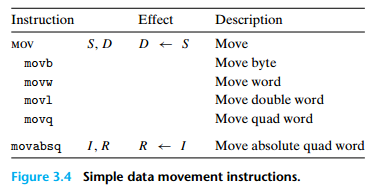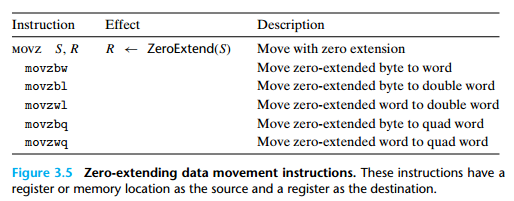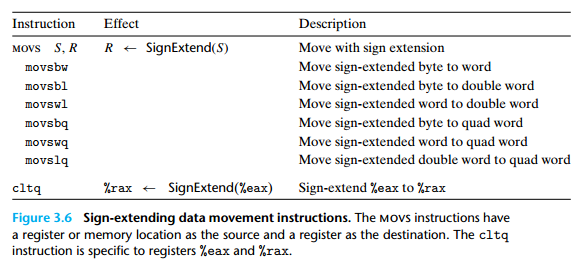【注】cltq 指令用于将 %eax 可符号扩展为 %rax，其不需要任何操作数。

• 栈操作指令类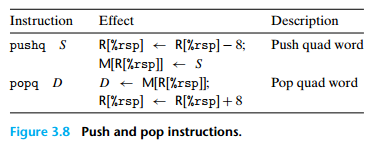• 算术逻辑运算指令类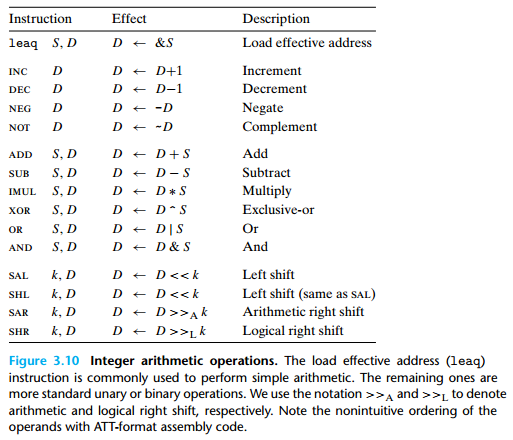【注】leaq 指令不设置条件码，因为它是用于进行地址计算的。对于逻辑操作，进位标志和溢出标志会设置位0。对于 INC 和 DEC 指令，不会设置 CF 位，因为该二者主要用于循环变量的加减，不修改 CF 位是考虑到循环中有可能进行高精度大数运算；而且 CF 位可以根据 ZF 位来判断，INC 且当前指令 ZF，则说明当前指令产生了进位；DEC 且上一条指令 ZF，则说明当前指令产生了借位。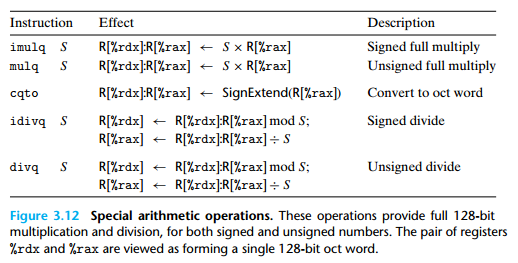【注】cqto 指令不需要操作数，隐含读出 %rax 的符号位并将它复制到 %rdx 的所有位。

• 比较和测试指令类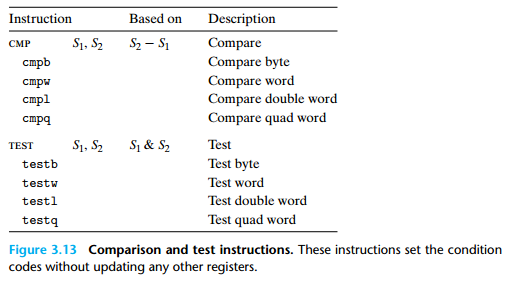【注】compqtestq 指令仅将计算结果用于设置条件码，而并不改变操作数。

• 条件传输指令类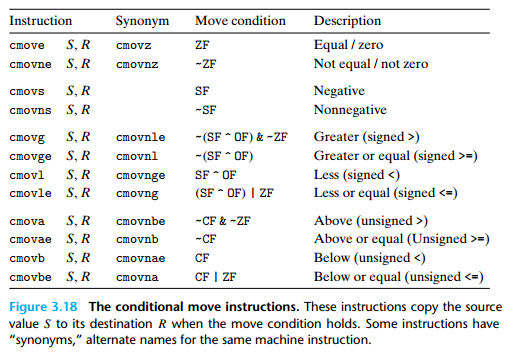• set 指令类
指令根据条件码组合将目的操作数的地位字节设置为 0 或 1，即满足设置条件时设为 1 ，不满足时设为 0 ，不改变其余字节。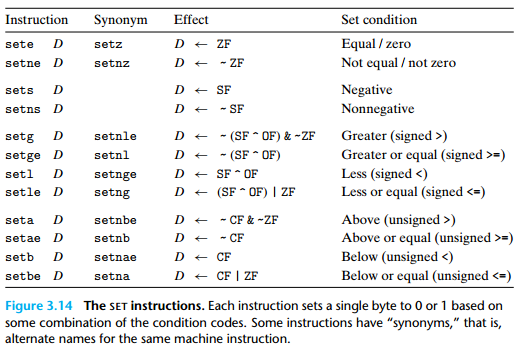• 跳转指令类
其中，jmp 为无条件跳转，其余为条件跳转。jmp 跳转分为直接跳转和间接跳转，直接跳转是跳转到标签对应的地址，间接跳转是跳转到寄存器或内存单元中存储内容值作为地址对应的位置。条件跳转只能是直接跳转。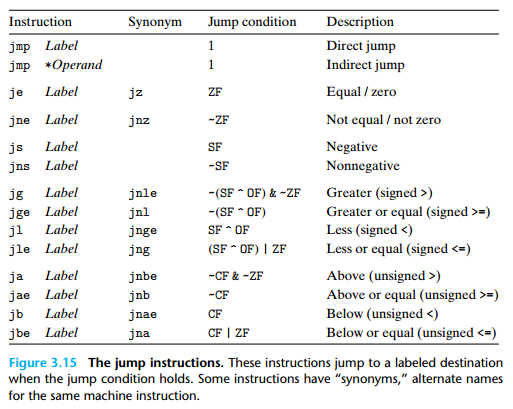### 2.4 寄存器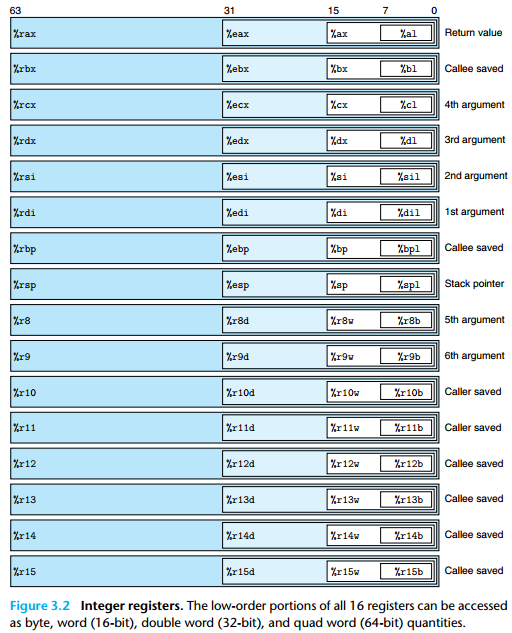• Linux 寄存器用法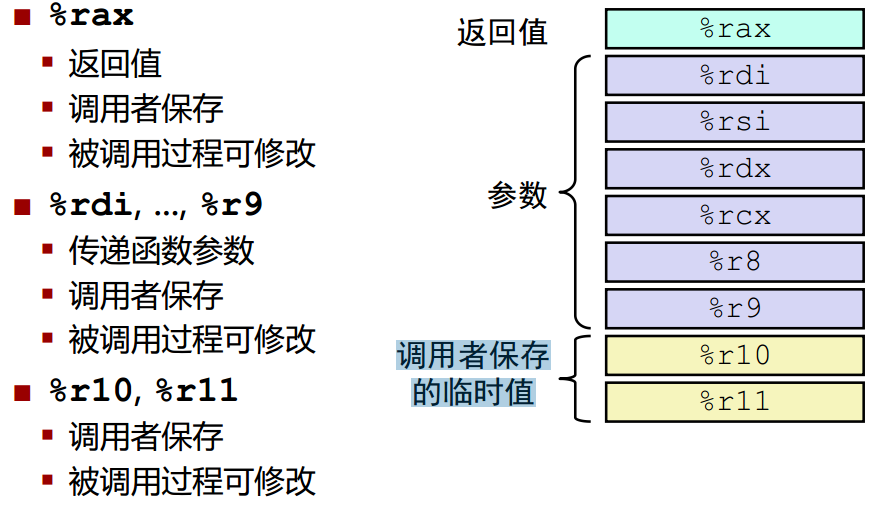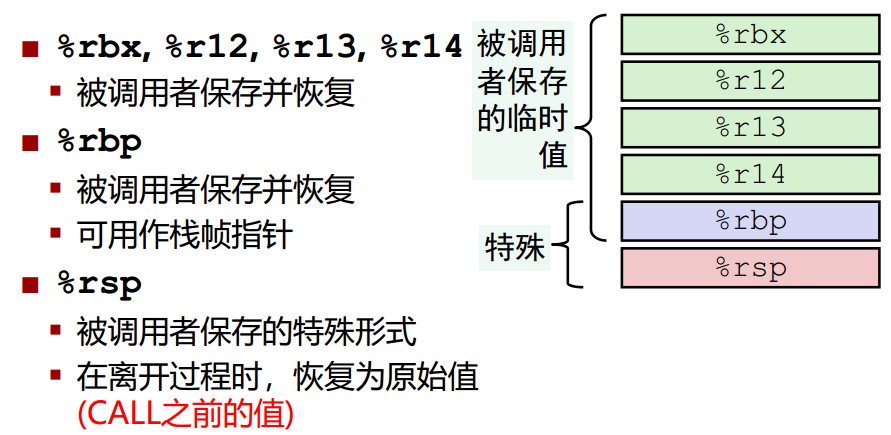#### 函数传参使用寄存器原则

• 输入参数
当函数的传入参数 <= 6 个时，使用寄存器传入；当 > 6 个时，1 ~ 6 个参数仍使用寄存器传参，第 7 个及后续参数使用堆栈传入。使用寄存器传入参数时规定参数对应的寄存器如下：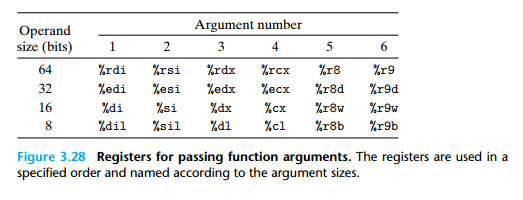• 输出参数
当函数的输出参数 <= 1 个时，使用寄存器 rax 传递输出参数，当输出参数 > 1 （比如结构体中包含多个字段）个时，使用堆栈传递参数，并且在 rax 中保留第一个输出参数作为返回。

### 2.5 程序结构

• do-while 语句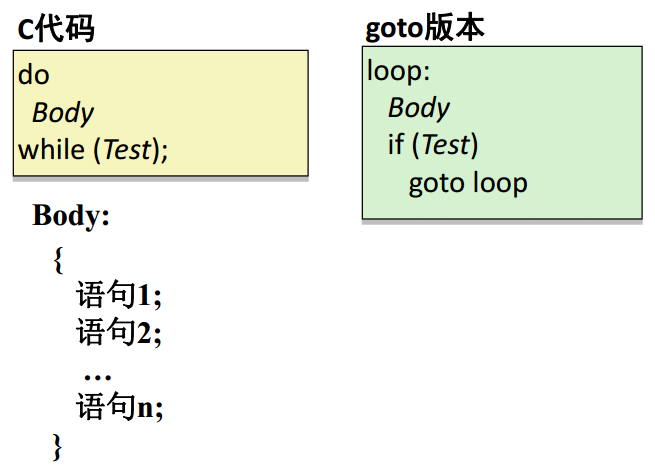• while 语句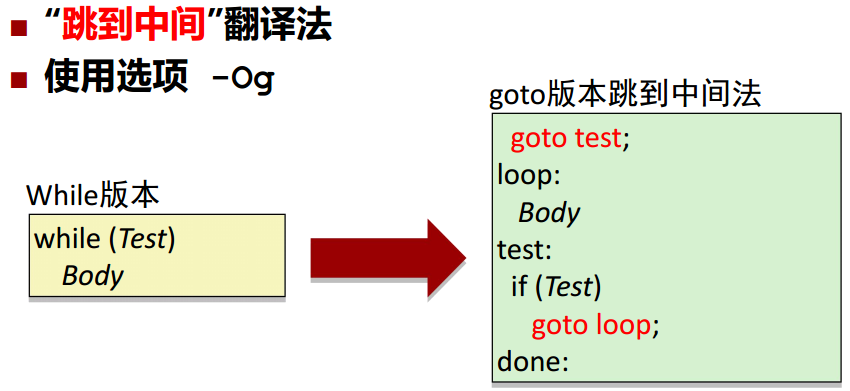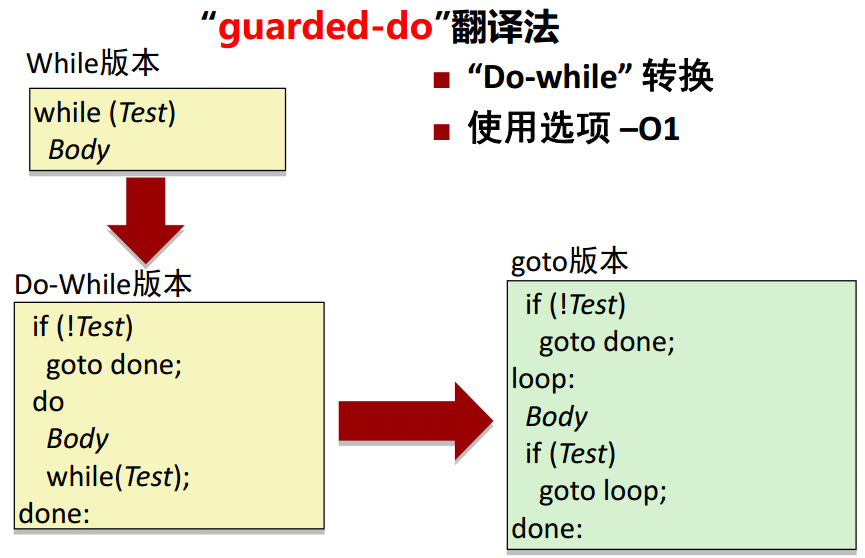• for 语句
通过转换为 while 语句或 do-while 语句实现。

• switch 语句

### 2.6 过程

• 栈结构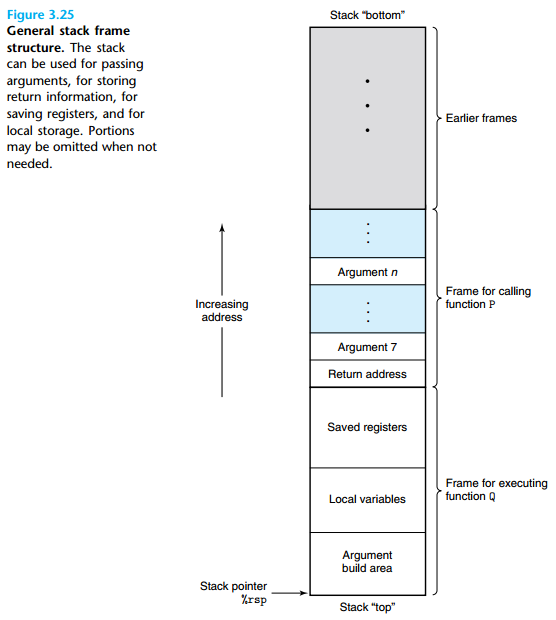• 过程数据流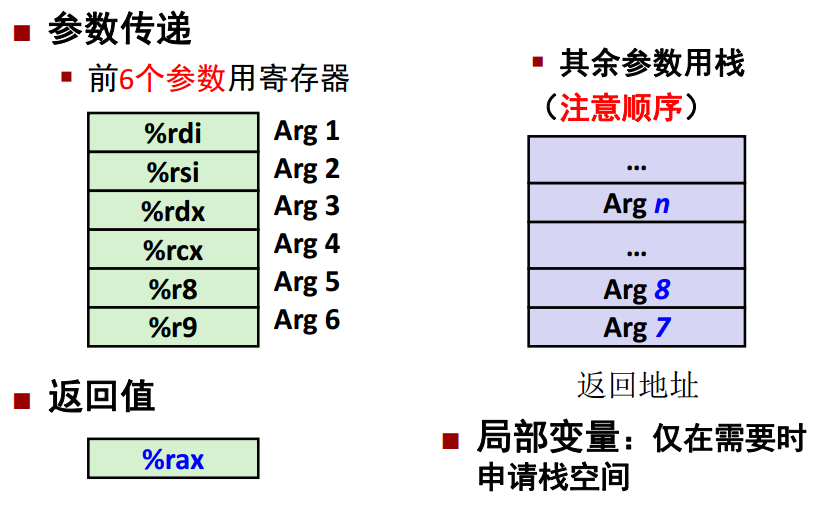### 2.7 指针和数组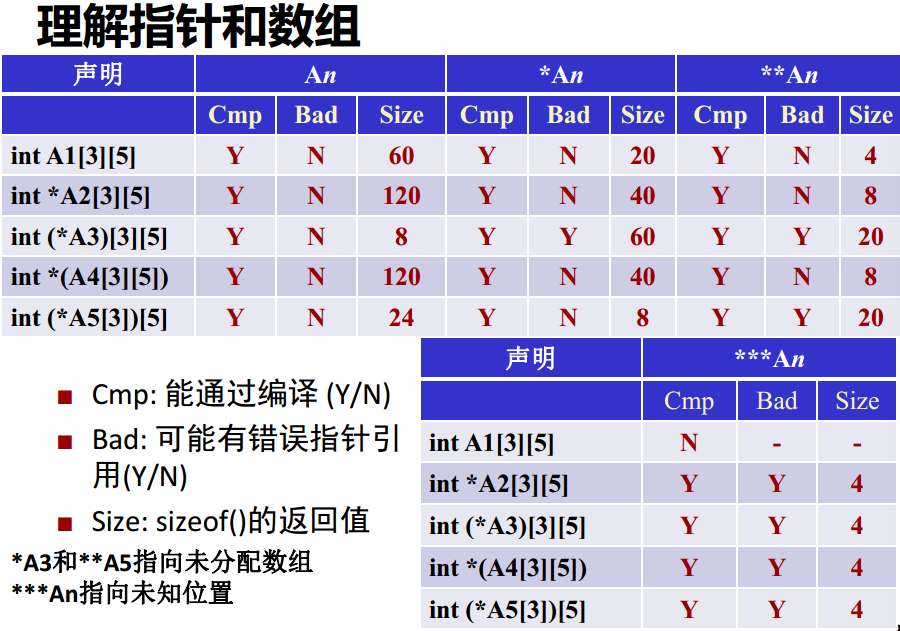### 2.8 结构体

• 结构体中的字段顺序必须与声明一致
• 每个结构体成员的偏移量是在编译阶段确定的

#### 对齐要求

1. 基本数据类型需要 K 字节
2. 每个成员偏移量地址必须是其数据类型 K 字节的倍数
3. 结构体的 K 是结构体中所有成员的 K 值最大值
• 结构体内部：满足每个元素的对齐要求
• 结构体外部：满足结构体整体对齐存放

### 2.9 缓冲区溢出

#### 对抗缓冲区溢出攻击

• 避免溢出漏洞：使用安全的库函数
• 使用系统级的保护：随机的栈偏移、非可执行代码段
• 栈金丝雀：设立并核对金丝雀

## 6. 存储器层次结构

### 6.1 高速缓存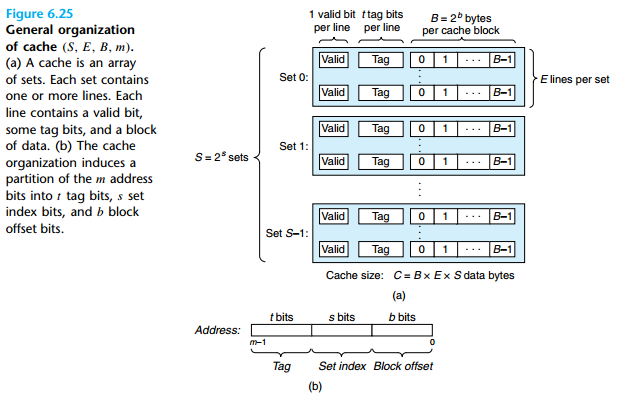$\begin{array}{c} C = S \times E \times B \end{array}$

#### 缓冲不命中

1. 冷（强制性）不命中：当缓存为空时， 对任何数据的请求都会不命中， 此类不命中称为冷不命中
2. 冲突不命中：冲突不命中发生在缓存足够大， 但是这些多个数据对象会映射到同一个缓存块
3. 容量不命中：发生在当活跃块集合（工作集 working set） 的大小比缓存大t） 的大小比缓存大